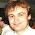## Sunday, August 28, 2011

### Testing MathJaxI want to ask all users who suddenly find this blog annoyingly slow because of Mathjax to complain in the comments here. If the number of complaints will exceed a certain threshold, I will disable Mathjax again. You see, the Mathjax javascript support is loading in the grey rectangles in the lower left corner...

Mathjax is the seventh web system for LaTeX I am testing on this blog. TeX - by Donald Knuth - or LaTeX - with some help from Leslie Lamport - is the experts' standard to write mathematical expressions and formulae - and texts with lots of formulae. Most papers at arXiv.org are originally written in TeX or LaTeX.

We clearly cannot afford to reserve $...$ for the inline mathematics because this blog is full of the dollar signs which usually denote the U.S. currency. However, we may be getting ready for the default of the U.S. government so if you fairly replace one dollar by three dollars, things will be fine. For example, $$E=mc^2$$$will appear as $$E=mc^2$$$.

Alternatively, you may use $$x^2+y^2=r^2$$ to type in-line maths, $$x^2 + y^2 = r^2$$.

Also, double dollars $$...$$ or the symbols $...$ are reserved for displayed equations. The resulting graphics looks like this:

$$\sum_{k=1}^n k = \frac{n(n+1)}{2}.$$

If you don't know how to type such nice equations, right-click at the equation and choose "Show source". It will show you how to type it. If you type it in between double dollars (displayed formula) or triple dollars (in-line maths), it will ultimately show up as pretty mathematics. Thank God, Mathjax respects the background color and the text color.

That's true even if the background is blue like in our "blockquote" format:
$$\sin^2 \psi + \cos^2 \psi = 1.$$\$
For you, the mathematical devices are available in the slow comments (slow feedback) and it will be so unless I will get enough feedback indicating that the people's computers and browsers are still too slow for the luxury of TeX or LaTeX to be classified as an irrational exuberance. ;-)

1.? nope

slow? Faster than light blog

2.Merde alors ! It seems impossible to download my avatar with my ipad... I only get a black picture. :-(

3.Test of separation of the Blogger, DISQUS comment systems after import, syncing turned on. Posted via Blogger mobile template.

4.Dear Shannon, please be patient. I also want to see your avatar etc. Can't you do it on a PC or Mac? Alternatively, I may create a DISQUS account for you that does include the Echo avatar and send you a password for the DISQUS account that you may change... Let me know. And if yes, let me know about the username that can't be the same one as a used one, but it may be a tiny variation.

5.Dear Lubos, you are very kind. I'll wait until I'm back home to my PC in a week. I still love my ipad who can feed me with my TRF addiction ;-)

6.hie! it works fine

7.(blockquote)
test
(/blockquote)

8.Dear Dimension10, DISQUS won't allow MathJax - or any injected script - for quite some time. It was pretty much a point of the 2012 revolution at DISQUS that they use iframes so all the scripts in the parent page are inconsequential for the DISQUS portion of the page.

9.No, I wrote "langle rangle" as in the "<>" "" notation works for the blockquotes.

10.Testing TESTING Testing Testing Testring ?